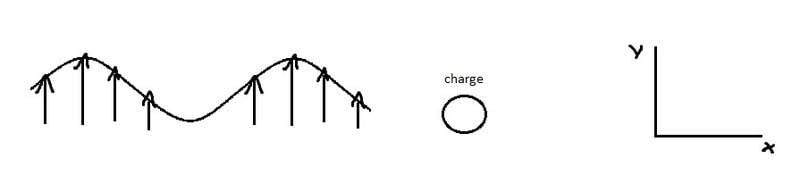# What causes light to bend in refraction

(Sorry my poor English.) How could we explain the bending of light after refraction? The wave in my example will cause the charge to move in the y direction. Now, analysing it from the particle point of view of light, since the momentum must be conserved, as the charge moves in a direction, the photon must travel in the opposite direction. Am I right?jfizzix
Gold Member
Refraction in a nutshell:

Light can be described as electromagnetic waves, which can be further quantized into "photons"

Electromagnetic (EM) waves push and pull charged objects according to the oscillating direction of the electric field at any given time.

A solid material can be described as a grid or lattice of atoms, with bound electrons surrounding each atom in a cloud. Sometimes some of these electrons are shared between all atoms, as in conductors, where electrons can move freely. When the electrons are not shared like this, the material is an insulator.

In electric conductors, light does not travel far into the material before being absorbed. Optical refraction is something we see mostly in insulators, like glass, which are not electrically conducting. If the light just gets absorbed, we can't see refraction anyway.

So, when an EM wave acts on these atoms, it pushes and pulls the electrons around them. Since accelerating charges emit their own EM waves, the total effect of the incident EM wave plus the induced EM wave adds up to an EM wave traveling at slower than c, the speed of light in vacuum. This speed is given by the index of refraction n=c/v, so that a material with index 3/2 implies that light traveling through it is going only at 2/3 c.

Light bends when encountering a material of different refractive index simply because light travels at a slower speed in that material. If the EM wave comes in at an angle different than straight on, then the wavefront on one side will take longer to reach the material surface than on the other side. This means that the difference in distances from the surface to one side of the wavefront, and to the other side of the wavefront will be smaller inside the material than outside the material; the direction of propagation given by those wavefronts is different between outside and inside, so the light bends.

Looking at momentum conservation, because the light bends in the material, the material experiences a kickback due to the change of momentum in light. This kickback is really, really small, but it is there. If the light exiting the material is going in a different direction than the light entering the material, momentum conservation requires that the material has gained some momentum from the light.

Surprisingly, there are still some open questions in current physics about refraction. In particular, if light travels more slowly in a material, does it have a smaller momentum because of smaller speed, or a larger momentum because of smaller wavelength? There's good theoretical results given, but experimentally measuring the momentum exchange for a pulse of light while it's inside a material hasn't been done yet, since it would be so hard to measure.

Thank you for clarifying that for me. Then what causes light to bend is the "delay" in the path of the wavelets when the wave arrives in the surface of the material. For the case where we have only one charge in the situation, as I described in the post 1#, is my reasoning correct?

jfizzix
Gold Member
It's hard for me to imagine how refraction works for a single atom.

The basic elements are there:
The wave pushes and pulls the charge
The accelerating charge produces a secondary wave

How the waves add together to give a delayed wave is unclear to me.

However, if you have a pulse of light incident on a single charge (say, an electron), the total momentum of the light plus the electron is conserved. After the pulse has interacted with the electron, the final momentum of the electron can be figured out from conservation of momentum, and knowing what the final momentum of both EM waves are.

•kent davidge